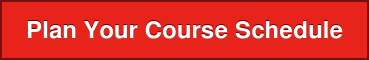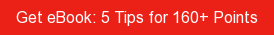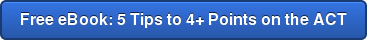# SAT / ACT Prep Online Guides and TipsOne great thing about the AP Physics 1 exam is that exam takers have access to a table of equations and formulas to reference during the exam (which is often referred to as the "AP Physics 1 equation sheet").

But the AP Physics 1 reference tables include a lot of information! If you aren't already familiar with the formula sheet before you take the exam, you might end up wasting valuable time trying to navigate the different equations and remember when and how to use them.

To help you out, we've developed a PrepScholar Physics 1 equation sheet. This sheet contains all the equations you'll see on the actual AP Physics 1 equation sheet plus additional explanations to help you use it as a study tool.

In the rest of this article, we're going to give you a detailed explanation of each table of information supplied on the AP Physics 1 equation sheet and explain how it can be used on the exam. We'll also give you three tips for using the formula sheet on the exam, and three tips for using the formula sheet to study for the exam.One of the things you'll see on the AP 1 Physics exam? Questions about electricity!

## The AP Physics 1 Exam

The AP Physics 1 Exam is an algebra-based exam that assesses exam takers' understanding of kinematics, dynamics, circular motion and gravitation, energy, momentum, simple harmonic motion, torque and rotational motion, electric charge and electric force, DC circuits, and mechanical waves and sound. Basically, the AP Physics 1 exam tests your understanding of the foundational concepts of classical mechanics!

This AP exam lasts for three hours and includes 50 multiple choice and five free response questions, with each section being worth 50% of the overall exam score. The multiple choice section is 90 minutes long, and the 50 questions on this part of the test are divided into two subsections. Here's how they break down:

 Section Number of Questions 1A 45 multiple choice questions 1B 5 multiple choice questions

The five free response questions last for 90 minutes, and the topic of each individual free response question is as follows:

 Question Number Question Topic/Format Question 1 Experimental Design Question 2 Qualitative/Quantitative Translation Question 3 Paragraph Argument/Short Answer Question 4 Short Answer Question 5 Short Answer

The AP Physics 1 equation sheet will be included in your exam booklet on the day of the exam, and you'll be able to use it for reference throughout the exam period.

Because there's so much to cover, we've put together a special, PrepScholar version of the equation sheet. It contains all the information you'll see on the original equation sheet plus explanations of each equation. We'll be using this equation sheet throughout the rest of the document, so be sure to download it now.

Next, we'll take a closer look at each table of information supplied on the AP Physics 1 formula sheet.This is the official AP Physics 1 Formula Sheet you'll receive on test day.

## The AP Physics 1 Equation Sheet Explained

The AP Physics 1 formula sheet is a key resource for answering questions on this algebra-based exam. A copy of the equations sheet will be provided in your exam booklet during the exam (you can't bring your own copies into the exam room), and it includes common equations that are covered throughout the AP Physics 1 course.

If you haven't already, make sure you download the PrepScholar equation sheet that you can use as a study tool. You'll be given a clean copy of the official study sheet on the day of the exam, but we recommend that you print off a copy that you can mark up and use as you study!

The AP Physics 1 equations sheet is built out into tables based on the following types of information:

• Constants and conversion factors (page 1)
• Unit symbols (page 1)
• Prefixes (page 1)
• Values of trigonometric functions for common angles (page 1)
• Equations commonly used in physics for mechanics, electricity, waves, and geometry and trigonometry (page 2)

The equations sheet is meant to help you quickly recall constants, conversion factors, symbols, prefixes, values, and equations that you may need in order to solve problems during the exam. It's important to keep in mind that each equation you use from the equations sheet must be supported by explanations and logical development in your answers on the exam. That means you'll have to actually understand the formulas and how to use them if you want to succeed on the AP Physics 1 test!

## How to Use the Formulas on the AP Physics 1 Equation Sheet

To help you get familiar with how to use the AP Physics 1 equations sheet, we'll break down how to use the following areas of the equation sheet individually. Specifically, we'll walk you through the following topics:

• Constants and conversion factors
• Prefixes and unit symbols
• Values of trigonometric functions
• Equations for mechanics, electricity, waves, and geometry and trigonometry.

Let's take a look at the major sections of the physics 1 equation sheet.### Constants and Conversion Factors

The constants and conversion factors appear at the top of the first page of the equations sheet that you'll use on the AP Physics 1 exam. These are fixed values that you will need to know and use in formulas and equations on the exam.

The constants and conversion factors supplied on the AP Physics 1 information sheet include proton mass, neutron mass, electron mass, speed of light, electron charge magnitude, Coulomb's law constant, universal gravitational constant, and acceleration due to gravity at Earth's surface.

So how will you use these conversion factors on exam day? The constants and conversion factors can be used on the exam to convert between one unit and another using multiplication or division. This will change the units of a measurement without changing the value of that measurement. The conversion factors provided on the equations sheet can be used to convert length, mass, time, energy, temperature, frequency, force, power, charge, and resistance.### Unit Symbols, Prefixes, and Values of Trigonometric Functions

The tables of unit symbols and prefixes can be combined to express values on the AP Physics 1 exam. The table of prefixes supplies the scientific notation, or factor, of a given prefix, the prefix, and the corresponding symbol.

That sounds confusing, but here's what we mean. For example, the prefix "tera" is supplied in the table, along with the correct factor of 1012, and the correct symbol, "T". Similarly, the table of unit symbols supplies the name of the unit and its correct symbol, such as "meter" and "m," or "kelvin" and "K".

The prefixes included on the information sheet are used when dealing with very large or small units in questions on the exam. The prefixes indicate specific powers of ten and will typically be used to express measurements, combined with a base word from the table of unit symbols (e.g. kilowatts, megajoules, etc.). This part of the table will help you better understand the questions on the exam, and they will help you double-check to make sure you're using the right units in your answers to the free response questions.

Finally, the values of trigonometric functions will be crucial when you use geometric and trigonometric equations to calculate the value of the angles of a right triangle. This table expresses the value of the most common angles (sin, cos, tan) at different degrees up to a 90 degree angle. You'll need to understand these in order to do things like analyze vectors!

One of the single most important parts of your college application is what classes you choose to take in high school (in conjunction with how well you do in those classes). Our team of PrepScholar admissions experts have compiled their knowledge into this single guide to planning out your high school course schedule. We'll advise you on how to balance your schedule between regular and honors/AP/IB courses, how to choose your extracurriculars, and what classes you can't afford not to take.### Equations

The second page of the AP Physics 1 formula sheet provided on the exam includes a list of common equations that you might need to use on the exam. The equations are divided up into four sections based on type: mechanics, electricity, waves, and geometry and trigonometry.

Below, we'll explain what types of problems the equations included in each section of the equations sheet will help you solve.#### The Mechanics Table

The equations in the Mechanics table can be used to calculate, describe, analyze, express, explain, and make claims and predictions about the following on the AP Physics 1 exam:

• Acceleration, including radial acceleration, tangential acceleration, and acceleration of an object interacting with other objects

• Motion, including linear motion, angular motion, and the motion of individual objects and two-object systems

• Force(s), including contact forces between objects such as tension, friction, normal, buoyant, and spring,

• Gravitational force, including the gravitational force that two objects exert on each other

• Gravitational force in various contexts

• Change(s) in kinetic energy, calculate total energy of a system, predict changes in the total energy of a system, calculate internal potential energy, calculate power

• Momentum, angular momentum, magnitude of angular momentum, change in angular momentum

• Torque#### The Electricity Table

The equations in the much shorter electricity table of the equations sheet can be used to calculate and describe the following on the AP exam:

• Magnitude of an electric field
• Conservation of electric charge
• Resistivity of matter
• Conservation of electric charge in electrical circuits#### The Wave Table

There is a single equation pertaining to waves on the AP Physics 1 formula sheet; this equation can be used to calculate the wavelength of a periodic wave.#### The Geometric and Trigonometric Equation Table

Finally, the last section of the equations sheet provides geometric and trigonometric equations that can be used to solve for the following:

• The area of a rectangle
• The area of a triangle
• The area and circumference of a circle
• The volume of a rectangular solid
• The volume and surface area of a cylinder
• The volume and surface area of a sphere
• The value of the angles of a right triangle

Since there are so many formulas and equations supplied in the AP Physics 1 reference tables, it's worth it to spend some time getting comfortable with them before you take the exam. We'll talk about the best ways to familiarize yourself with the contents of the equation sheet below.## 3 Tips for Using the AP Physics 1 Formula Sheet as a Study Guide

Since the AP Physics 1 reference tables will be available to you on the actual exam, you can take advantage of having this resource ahead of time by using it to study for the exam. Check out our three tips for studying with the AP Physics 1 formula sheet below!

### Study Tip 1: Make Flashcards of the Equations

It's pretty much guaranteed that the equations provided in the AP Physics 1 formula sheet will appear on the exam. Though you'll have these equations at your fingertips while you're taking the exam, you don't want to waste valuable exam time trying to decode them.

The equations sheet provides a symbol key to help you decode what each symbol in a given equation stands for, but you'll have more time to answer exam questions accurately if you don't have to use that part of the exam sheet throughout the exam.

Instead, to study for the exam, use the AP Physics 1 equations sheet to create flashcards that will help you memorize the equations. In order to use the equations sheet to your advantage while you study, your flashcards should each have an equation from the equations sheet on one side, and a key that breaks down each variable in the equation on the opposite side. If you already know what "v," or "K," or "U" stands for in each equation on the exam, you won't have to spend time using the equations sheet to break down every variable in an equation that you need to use to solve a problem.

Looking for help studying for your AP exam?

Our one-on-one online AP tutoring services can help you prepare for your AP exams. Get matched with a top tutor who got a high score on the exam you're studying for!### Study Tip 2: Take a Practice Test

Probably the best way to get a feel for how best to use the AP Physics 1 formula sheet on the actual exam is to take a practice test — or at least do a series of practice questions — using the sheet as a resource.

While there aren't many AP Physics 1 practice exams available, there is an unofficial one available from CrackAP. You can also work through the FRQs from past exams, which you can find on the College Board website.

As you practice, consider noting when you most need to reference the equations sheet, then spend some extra time studying the notes or flashcards you have that pertain to those areas. This will help you identify your weaker areas and strengthen them before you take the AP exam.

### Study Tip 3: Memorize the Layout

If you get to the exam and aren't already familiar with the layout of the equations sheet and what equations are and aren't included, it's going to be pretty difficult to use the sheet to your advantage during the exam.

Taking some time to memorize which information is located where on the equations sheet, and having a general sense of what equations and information are included on the sheet will help you know exactly when you can turn to the equations sheet for information or reminders as you take the exam. Memorizing the information sheet will help you be more efficient and organized when you take the AP Physics 1 exam.Like we've already said—the best use of the AP Physics 1 equation sheet is to help you learn important equations you'll need to know on test day. But just in case you didn't, here are our best tips for using the sheet on the actual exam.

## 3 Tips for Using the AP Physics 1 Equation Sheet on Exam Day

Since you're allowed to use the CollegeBoard's PDF of the AP Physics formula sheet on exam day, you want to make sure you know how to use the sheet to your advantage while you're actually taking the exam. Read on for our three tips for using the AP Physics formula sheet on exam day!

### Tip 1: Save Yourself Time

Since the AP Physics 1 exam is timed, you really don't want to spend more time than is absolutely necessary trying to remember values, formulas, and equations during the exam. If you get stuck and just can't remember a value or portion of an equation that is crucial to answering a question, quickly flipping to the equations sheet can help jog your memory.

### Tip 2: Make Quick Conversions

The constants and conversion factors that are commonly used in physics problems are a little tricky. They typically include multiple decimal points, exponents, and other symbols that can be difficult to memorize before the exam. The equations sheet will be helpful for quickly making conversions and remembering the correct expressions for common constants as you solve problems on the test.

### Tip 3: Check Your Work

When answering questions on the AP Physics 1 exam, attention to detail is crucial. But this can be difficult on a timed test, and it's likely easier to accidentally forget to include a symbol, exponent, or notation than you might think. Saving a few minutes to check over your work using the equations sheet during the exam can help you make corrections and ensure that you've written formulas and equations out correctly, particularly on the free response questions.## What's Next?

This article covers the Physics 1 equation sheet, but did you know that you can take two other AP Physics courses while you're in high school? Get the scoop on AP Physics 1, 2, and C — and the differences between them.

If you're looking for resources for IB Physics courses, we've got those, too. Here's an expert guide to the IB Physics syllabus. We've also compiled a list of the best study notes for Physics SL and HL.

You may be wondering how hard AP Physics 1 really is. To get the answer, check out this article that will help you figure out what the hardest AP classes are for you.

Want to improve your SAT score by 160 points or your ACT score by 4 points? We've written a guide for each test about the top 5 strategies you must be using to have a shot at improving your score. Download it for free now:Ashley Robinson

Ashley Sufflé Robinson has a Ph.D. in 19th Century English Literature. As a content writer for PrepScholar, Ashley is passionate about giving college-bound students the in-depth information they need to get into the school of their dreams.

Student and Parent Forum

Our new student and parent forum, at ExpertHub.PrepScholar.com, allow you to interact with your peers and the PrepScholar staff. See how other students and parents are navigating high school, college, and the college admissions process. Ask questions; get answers.